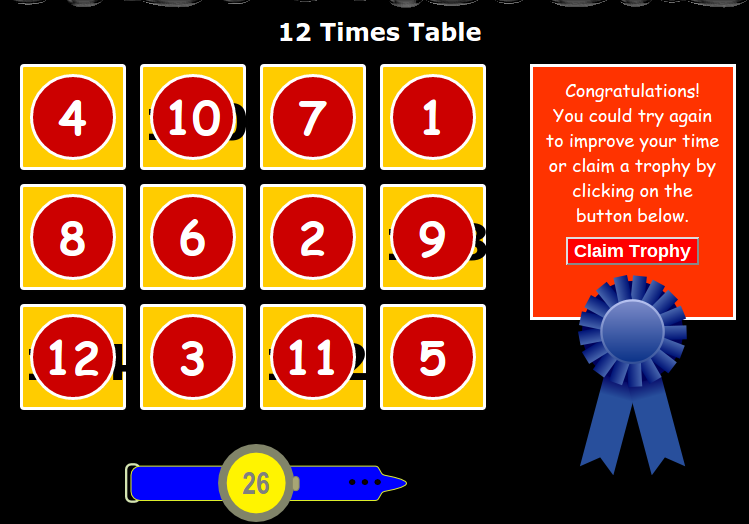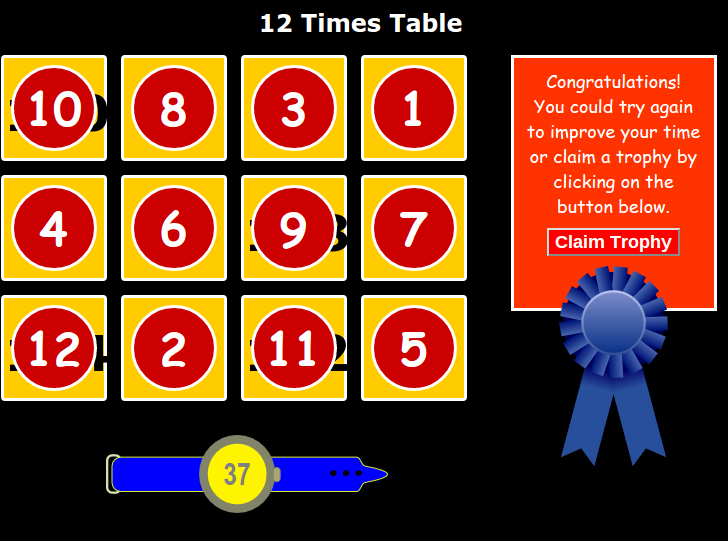## Monday, 25 May 2015

### Saruja- 13x Table Practise

12x Table

 1 x 12 = 12 2 x 12 = 24 3 x 12 = 36 4 x 12 = 48 5 x 12 = 60 6 x 12 = 72 7 x 12 = 84 8 x 12 = 96 9 x 12 = 108 10 x 12 = 120 11 x 12 = 132 12 x 12 = 144 1 x 12 = 12 2 x 12 = 24 3 x 12 = 36 4 x 12 = 48 5 x 12 = 60 6 x 12 = 72 7 x 12 = 84 8 x 12 = 96 9 x 12 = 108 10 x 12 = 120 11 x 12 = 132 12 x 12 = 144 1 x 12 = 12 2 x 12 = 24 3 x 12 = 36 4 x 12 = 48 5 x 12 = 60 6 x 12 = 72 7 x 12 = 84 8 x 12 = 96 9 x 12 = 108 10 x 12 = 120 11 x 12 = 132 12 x 12 = 144 1 x 12 = 12 2 x 12 = 24 3 x 12 = 36 4 x 12 = 48 5 x 12 =  60 6 x 12 = 72 7 x 12 = 84 8 x 12 = 96 9 x 12 = 108 10 x 12 = 120 11 x 12 = 132 12 x 12 = 144 1 x 12 = 12 2 x 12 = 24 3 x 12 = 36 4 x 12 = 48 5 x 12 = 60 6 x 12 = 72 7 x 12 = 84 8 x 12 = 96 9 x 12 = 108 10 x 12 = 120 11 x 12 = 132 12 x 12 = 144 1 x 12 = 12 2 x 12 = 24 3  12 = 36 4 x 12 = 48 5 x 12 = 60 6 x 12 = 72 7 x 12 = 84 8 x 12 = 96 9 x 12 = 108 10 x 12 = 120 11 x 12 = 132 12 x 12 = 144 1 x 12 = 12 2 x 12 = 24 3 x 12 = 36 4 x 12 = 48 5 x 12 = 60 6 x 12 = 72 7 x 12 = 84 8 x 12 = 96 9 x 12 = 108 10 x 12 =120 11 x 12 = 132 12 x 12 = 144 1 x 12 = 12 2 x 12 = 24 3 x 12 = 36 4 x 12 = 48 5 x 12 = 60 6 x 12 = 72 7 x 12 = 84 8 x 12 = 96 9 x 12 = 108 10 x 12 = 132 11 x 12 = 132 12 x 12 = 144 1 x12= 12 2 x 12 =24 3 x 12 =36 4 x 12 = 48 5 x 12 = 60 6 x 12 = 72 7 x 12 = 84 8 x 12 = 96 9 x 12 = 108 10 x 12 = 120 11 x 12 = 132 12 x 12 = 144 1 x 12 = 12 2 x 12 = 24 3 x 12 = 36 4 x 12 = 48 5 x 12 = 60 6 x 12 = 72 7 x 12 = 84 8 x 12 = 96 9 x 12 = 108 10 x 12 = 120 11 x 12 = 132 12 x 12 = 144Today for our math we did multiplication practice. We have to choose one times table for our week and practise it and when we finish practise this game. We did this game to see that if we are good at multiplication, if are not we have to learn it.  I already know my 1,2 ,3 ,4 ,5 ,6 ,7 ,8 ,9 ,10,11 and now I am learning 12 times table. I think I did my best and I can do faster than this time.